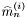#Boost C++ Libraries

...one of the most highly regarded and expertly designed C++ library projects in the world.

## Struct template weighted_skewness_impl

boost::accumulators::impl::weighted_skewness_impl — Skewness estimation for weighted samples.

## Synopsis

```// In header: <boost/accumulators/statistics/weighted_skewness.hpp>

template<typename Sample, typename Weight>
struct weighted_skewness_impl {
// types
typedef numeric::functional::multiplies< Sample, Weight >::result_type                weighted_sample;
typedef numeric::functional::average< weighted_sample, weighted_sample >::result_type result_type;

// construct/copy/destruct
weighted_skewness_impl(dont_care);

// public member functions
template<typename Args> result_type result(Args const &) const;
};```

## Description

The skewness of a sample distribution is defined as the ratio of the 3rd central moment and the-th power \$ of the 2nd central moment (the variance) of the samples. The skewness can also be expressed by the simple moments:

Equation 1.29.whereare the-th moment andthe mean (first moment) of thesamples.

The skewness estimator for weighted samples is formally identical to the estimator for unweighted samples, except that the weighted counterparts of all measures it depends on are to be taken.

### `weighted_skewness_impl` public construct/copy/destruct

1. `weighted_skewness_impl(dont_care);`

### `weighted_skewness_impl` public member functions

1. `template<typename Args> result_type result(Args const & args) const;`